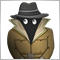How does iMACD MODE_SIGNAL work?22

Hi all,

I am pretty new to forex and MQL4, I tried to find an answer to my question using google and the search button but didn't find anything useful.

My question is, what value does MODE_SIGNAL give when using iMACD?

The reason why I am asking is that I am comparing those values, to the one that MACD_Histogram (that I have by default as part of MT4) plots on screen.

MACD_Histogram draws 2 lines, a blue and a red one.

When I use iMACD MODE_MAIN, the value matches the blue line.

When I use MODE_SIGNAL, the value doesn't match any of the MACD_Histogram values

For example, using EURUSD on H4 (2019.05.17 20:00), on the indicator I get -0.001191 on the blue and -0.000842 on the red.

Using iMACD I get -0.001191 on MODE_MAIN and -0.000807 on MODE_SIGNAL

I can calculate the signal value to match the indicator (it comes with the source code), which is probably what I will do, but I would like to understand what I am missing.

Thanks a lot12304

iMACD There may be different parameters? MODE_SIGNAL is drawn in different ways.22

in the MACD_Histogram Signal is calculated with this formula:

SignalLineBuffer[i] = alpha*MACDLineBuffer[i] + alpha_1*SignalLineBuffer[i+1];

with:

alpha = 2.0 / (SignalMAPeriod + 1.0);

alpha_1 = 1.0 - alpha;

The modes I can use are only 0-MODE_MAIN, 1-MODE_SIGNAL, so not sure what I can change there. In the notes it says that somewhere it's called MACD histogram, that's why I was assuming it would give the same values139429

Tucaninho:

in the MACD_Histogram Signal is calculated with this formula:

SignalLineBuffer[i] = alpha*MACDLineBuffer[i] + alpha_1*SignalLineBuffer[i+1];

with:

alpha = 2.0 / (SignalMAPeriod + 1.0);

alpha_1 = 1.0 - alpha;

The modes I can use are only 0-MODE_MAIN, 1-MODE_SIGNAL, so not sure what I can change there. In the notes it says that somewhere it's called MACD histogram, that's why I was assuming it would give the same values

That is simply EMA of MACD12304

Use iCustom for a custom indicator.